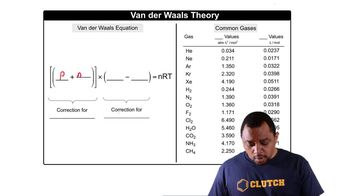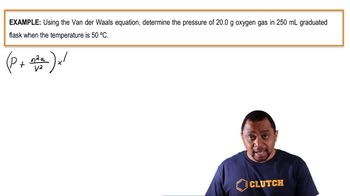Start typing, then use the up and down arrows to select an option from the list.
7:54 minutes
Problem 94a
Textbook Question

# Calculate the pressure that CCl4 will exert at 80 °C if 1.00 mol occupies 33.3 L, assuming that (a) CCl4 obeys the ideal-gas equation (b) CCl4 obeys the van der Waals equation. (Values for the van der Waals constants are given in Table 10.3.)Verified Solution
This video solution was recommended by our tutors as helpful for the problem above.
101views

### Watch next

Master Van der Waals Equation with a bite sized video explanation from Jules Bruno

Start learning01:4003:1903:55